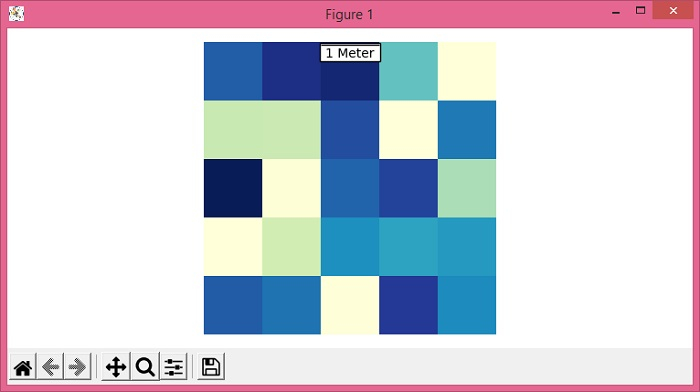# How to insert a scale bar in a map in Matplotlib?

MatplotlibPythonData Visualization

To insert a scale bar in a map in matplotlib, we can use AnchoredBar() class to instantiate the scalebar object.

## Steps

• Set the figure size and adjust the padding between and around the subplots.

• Create random data using numpy.

• Use imshow() method to display data as an image, i.e., on a 2D regular raster.

• Get the current axis using gca() method.

• Draw a horizontal scale bar with a center-aligned label underneath.

• Add a scalebar artist to the current axis.

• Turn off the axes.

• To display the figure, use show() mthod.

## Example

from matplotlib import pyplot as plt
from mpl_toolkits.axes_grid1.anchored_artists import AnchoredSizeBar
import numpy as np

plt.rcParams["figure.figsize"] = [7.50, 3.50]
plt.rcParams["figure.autolayout"] = True

data = np.random.rand(5, 5)

img = plt.imshow(data, cmap="YlGnBu")
ax = plt.gca()
scalebar = AnchoredSizeBar(ax.transData, 1, "1 Meter", 9)

plt.show()